# FORGE Course

|   |   |

## Congestion Control

TCP is used for reliable transport of data in the Internet. We previously study connection management and TCP mechanisms. In the following exercises, we will get interest in a other fundamental behavior of TCP: the congestion control.

## Congestion detection

TCP was designed at the end of the 70's. Several congestion control algorithms have been added since, mainly following the work of Van Jacobson published in 1988. They continue to evolve in different TCP variants. The exercises proposed in the following are founded on the last versions: RFC 5681 of September 2009.

1. For TCP, which phenomenon indicates congestion in the network?

2. What's going on inside a router to generate this phenomenon?

3. For TCP, this phenomenon can infer congestion. But it can also occur when there is no congestion in the network. In which other cases in which case such a phenomenon may occur?

4. If this phenomenon does not always indicate congestion, why is TCP based on this inference? Why don't we use an approach where the router notify explicitly the congestion by sending a message to the sender?

## Congestion control algorithms

For congestion control, TCP uses a threshold that indicates the flow rate above which congestion may occur. This threshold is expressed by the parameter ssthresh (in bytes). To get the flow rate threshold, ssthresh is divided by the RTT (Round Trip Time). The flow rate can vary from below and above the threshold ssthresh/RTT. The issuer maintains an other parameter, cwnd (Size of the congestion window), which indicates the maximum number of bytes it can send before receiving an acknowledgment. When cwnd > ssthresh, the sender take care particularly to not cause congestion.

1. Suppose ssthresh is at 5000 bytes, cwnd is at 6000 bytes, and segment size is 500 bytes. The sender sends twelve segments of 500 bytes in one RTT period, and receives twelve acknowledgements (one for each segments). What happens to the values ?ssthresh and cwnd? How these values changes are called?

2. Suppose ssthresh is still at 5000~bytes, cwnd is now at 14,000~bytes, the sender sends 14.000/500 = 28 segments, and that the sender receives a congestion notification before receiving the first acknowledgement. What happens to the ssthresh and cwnd values ? How these values changes are called?

3. We have seen how increases and decreases cwnd depending on the absence or presence of indicators of congestion. How do we call this algorithm? On what principle is based this algorithm?

4. At startup, and after having received a congestion notification, the value of cwnd is smaller than the value of ssthresh. Describe how cwnd increase when it is lower than ssthresh, depending on the following example. Suppose ssthresh equal to 3000 bytes and cwnd equal to 500 bytes, the size of a segment. The transmitter has several segments ready to be sent. How many segments sends the issuer during the first RTT period? If it receives acknowledgments for all segments, what becomes the value of cwnd? How many segments sends the issuer during the second RTT period? If it receives acknowledgments for all segments, what becomes the cwnd value? In general, how evoluate the size of cwnd?

5. How is called the period during which cwnd is smaller than ssthresh?

6. What happens to the value ssthresh if the sender receives a congestion notification while cwnd is smaller ssthresh that?

## Average bandwidth of a TCP connection

Suppose we wish to perform a large data transfer through a TCP connection

1. By neglecting the period during which cwnd is smaller than ssthresh, show that d, the average flow rate associates to a TCP connexion, is equal to: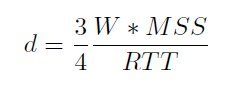where W is the size of the window (in segments) at the time of congestion, MSS the size of segment (assumed to maximum), and RTT is the round trip delay (assumed constant during the period of transmission).

2. Show that the loss rate p is equal to: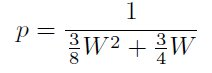3. Show that if the loss rate observed by a TCP connection is p, then d, the average flow rate, could be approximated by: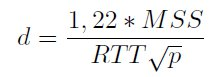4. What other parameters can affect the throughput of a TCP connection?

5. What utility do you see to the relation calculated in the last formula of d?

## Study of the latency of a web server

We would like to study the latency bound to the answer to an HTTP request. We make the following simplifying assumptions:

• The network is not congested (no losses or retransmissions);

• The receiver has infinite reception buffer (transmitter only limitation is due to the congestion window)

• The size of the object to receive the server is O, an integer multiple of MSS (MSS size is S bits);

• Throughput of the link connecting the client to the server is R (bps) and we neglected the size of all headers (TCP, IP and Link layer). Only the segments carrying data have a significant transmission time. The transmission time of control segments (ACK, SYN ...) is negligible;

• The value of the initial threshold of congestion control is never reached;

• The value of the round trip time delay is RTT.

1. Initially, we assume that we have no congestion control window. In this case, justify the following expression of L, the latency:2. We now assume a static congestion window of fixed size fixe W. Calculate the latency in this first case: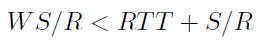3. We still assume a static congestion window of fixed size W. Calculate the latency in the second cases: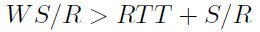4. Compare the latency with a dynamic congestion control window (slow start) with the one without congestion control.

## Analysis of TCP mechanisms

In this part you will generate traffic to a distant server using the PlanetLab Europe testbed. For each one, draw the chronogram and study the congestion control mechanisms put-in-work. Discuss particular of the following

1. What is the average RTT ?

2. Do you recognize the congestion control mechanismes ?

3. Up to how many segments are transmitted by RTT ?

4. What is the average throughput achieved then ?

5. A continuous sending it appears ?

6. Is there any disturbances (desequencement, retransmission...) ?